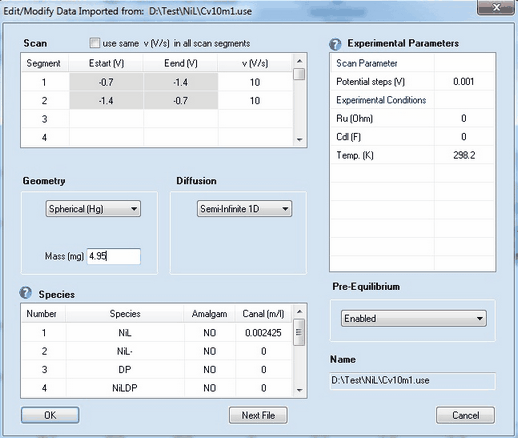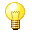﻿ Menu Command: Edit/Modify
DigiElch Help

# Menu Command: Edit/Modify# Menu Command: Edit/ModifyThis command opens a dialog box that enables the user to view (or modify) the parameters imported from an experimental data file.## 1. Scan Parameters:

Scan segment, v (V/s)
Starting potential, Estart (V), and end-potential, Eend (V), are extracted from the imported current-voltage couples and cannot be modified. The parameter, v (V/s), is the scan rate. The parameter can be modified for the reasons explained in Scenario 1.

Check Box: use the same value of v(V/s) in each scan segment
The possibility that an individual scan rate can be defined for each scan segment leads inevitably to more inconvenience, if, for instance, the scan rate in a CV composed of 20 scan segments with equal scan rate must be changed. For this reason, this option can be disabled if only "classical" CVs are simulated. In the latter case, any modification of the scan rate will be taken over for all other scan segments if the checkbox use the same value of v(V/s) in each scan segment is activated.

Check Box: apply background correction
This checkbox is visible only if both Ru (Ohm) and Cd (F) are different from zero. Click here for further details.

Potential steps (V):
The value is extracted from the imported current-voltage couples. If the value is modified, the imported current curve is replace by a cubic-spline interpolation using the entered potential steps. The dilution of data might be useful for saving computation time when using such files in a fitting project.

## 2. Pre-Equilibrium

Disabled
the simulation starts using the analytical concentrations, i.e. Cinit = Canal for each species.

Enabled
all equilibrium constants (chemical and electrochemical ones) entered on the Property Page: Chemical Reactions are used to compute the equilibrium distribution of all species from the analytical concentrations. The equilibrium values are used as initial concentration Cinit when starting the simulation. This option may lead to unexpected results as the quiet time before starting an experiment is usually not large enough to accomplish that the equilibrium distribution predicted by the Nernst equation is obtained in the entire diffusion layer.

Smart
The relation between Estart (V) and the standard potentials, Eo (V), decides how the analytical concentrations are assigned to the oxidized or reduced form of a redox couple. The initial concentrations are computed then in a second step taking only the chemical reactions into consideration. This option is optimal for all practical purposes unless the user is going to simulate a CV using Estart (V) not quite different from Eo (V).

## 3. Diffusion

Semi-Infinite 1D
diffusion is treated in one space direction either by making use of symmetry properties of the electrode or by neglecting contributions from other space directions. The user should be aware that this approach may lead to a considerable error in the current density when approximating a "small" planar (two-dimensional) electrode in terms of an infinitely large (effectively one-dimensional) electrode.

Finite 1D
simulation is executed for a thin layer electrode. When selecting this mode, a dialog field appears that enables the user to enter the Thickness of the thin layer electrode while and the right-hand boundary condition can be selected on the Property Page: Chemical Reactions.

Semi-Infinite 2D
diffusion is simulated in two space directions. This option is much more time consuming because diffusion occurring parallel to the electrode surface is not neglected when using this option.

## 4. Geometry

Depending on the selected option for Diffusion the following geometry options are available

 diffusion mode electrode geometry input parameter 1 input parameter 2 Semi-Infinite 2D Band electrode Width (cm) electrode Length (cm) " Disk electrode Radius (cm) none Finite 1D Planar electrode Area (cm²) none Semi-Infinite 1D Planar electrode Area (cm²) none " Spherical (Ro) electrode Radius (cm) none " Spherical (Hg) Mass (mg) of mercury drop none " Hemispherical electrode Radius (cm) none " Cylindrical electrode Radius (cm) electrode Length (cm) " Hemicylindrical electrode Radius (cm) electrode Length (cm)

When selecting Spherical (Hg) the user has the choice to specify whether a particular species is going to form an amalgam (i.e. diffusing into the mercury drop) or not.

## 5. Experimental Conditions

Ru (Ohm), Cdl (F)
Uncompensated ohmic resistance of the solvent and double layer capacity of the electrode which are to be included into the simulation. Cdl is assumed to have a constant value independently of the potential. Such an ideal behavior cannot be expected to hold true in real electrochemical measurements. Hence, when fitting experimental data the effect of both parameters should be preferably eliminated by using IR-compensation and subtracting the background currents.

Temp (K)
The temperature entered in this field is exclusively used for computing the effect of the Nernst-factor. Since DigiElch has no special knowledge about the temperature dependence of thermodynamic and kinetic parameters involved in a particular reaction scheme, it should be obvious, that such information cannot be obtained by executing a series of simulations referring to different temperatures !

Why do I need to modify imported data?

The option for modifying experimental parameters may be useful (or even necessary) for the following reasons:

Scenario 1: Modifying experimental data imported from ASCII-files which are not compliant with DigiElch's use-file format

ASCII-files (generated by third-party electrochemical hardware) which are not compliant with DigiElch's use-file format do usually not store all relevant experimental conditions in the ASCII file. In many cases, only the experimentally measured CV is stored in the form of voltage-current couples. If the user wants to use such files in a data fitting project, DigiElch needs to know to which experimental parameters (such as scan rate, electrode size and geometry, diffusion modus, IR-drop, analytical species concentrations etc.) each individual experimental CV refers. This can be done by applying the Edit/Modify command and entering the correct parameters for each imported curve in the dialog box shown on the top of this page. In many cases, there is only one parameter (such as the scan rate) that varies from experiment to experiment while other parameters (such as electrode size and geometry or species concentrations) are constant but not stored in the ASCII-file. In order to avoid that non-varying parameters need to be repeatedly entered for a great number of imported files, the following rule applies:when importing data from ASCII-files, the scan-, pre-equilibrium-, diffusion- and geometry-parameters as well as the experimental conditions entered for the active simulation (in the Tabbed Window: Simulations) will be transferred to the imported experiment.

Scenario 2: Modifying experimental data imported from use-files

The DigiElch use-file format contains a full description of the parameters (such as scan rate, electrode size and geometry, diffusion modus, IR-drop, analytical species concentrations etc.) to which the experiment refers. In other words, if all parameters have been correctly exported to the use-file, any modification after re-importing the file will result in a falsification. Nevertheless, such a falsification may be useful, for instance, for studying how parameter errors (such as a neglected IR-drop or the neglected edge effect when approximating a two-dimensional electrode by a planar one)  is passed to the diffusion coefficient of a particular species or to the heterogeneous rate constant of  the redox couple.